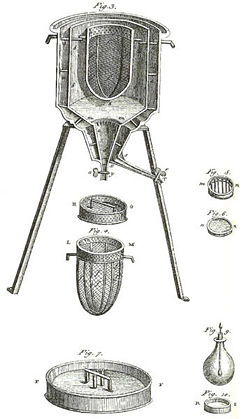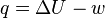CalorimeterThe world’s first ice-calorimeter. Antoine Lavoisier and Pierre-Simon Laplace used it in the winter of 1782-83, to determine the heat produced during various chemical changes. These experiments marked the founding of the field of thermochemistry.

A calorimeter is an instrument used for measuring the quantity of heat absorbed or released by matter when it undergoes a chemical reaction or physical change. Historically, such precision measurements have helped open a window onto the molecular and atomic structure of matter because the movement of molecules and atoms in matter collectively carries a quantity of heat energy that is distinctive for each type of matter and its chemical reactions. Based on such calorimetric measurements, scientists have developed tables giving the heat capacities of substances. Data produced by calorimeters has been foundational to the development of such technologies as steam boilers, turbines, rocket engines, internal combustion engines, oil refineries, and plastic product factories.

A widely accepted standard reference material used in many calorimeters is water because it has a high and precisely measured heat capacity, is easy to work with, and is readily available. A simple type of calorimeter would consist of an insulated container of water with a thermometer fixed in the water. To measure the heat capacity of molten lead, for example, an investigator could place a bowl of molten lead inside the calorimeter and record the temperature drop of the cooling lead and the corresponding temperature rise of the water. Knowing the mass of both the water and the lead, the investigator would have enough information to calculate lead's heat capacity.

Calorimeters come in many different types, some targeted to measuring the heat capacity of new materials (differential scanning calorimeters), while others measure such diverse aspects as the heat generated by new or untested reactions (isothermal microcalorimeters), heat of combustion and burn rates (accelerated rate calorimeters), and the energy of elementary particles (electromagnetic and hadronic calorimeters).

The word "calorimeter" is derived from the Latin word calor, meaning heat. The method or process of carrying out such measurements is called calorimetry.

Representative calculations

Calculating the heat capacity of molten lead based on data collected in the example above requires using the formula

Q = smΔT

where Q is the quantity of heat, s is the specific heat(the material's heat capacity divided by the heat capacity of water), m is the mass of the material, and ΔT is the temperature change. Inside the calorimeter, the heat lost by the lead(Ql) is, to the first approximation, equal to the heat gained by the water(Qw]). In this case, (smΔT)l must be equal to (smΔT)w. Since the only unknown quantity here is sl, it can be calculated according to the formula

sl = (smΔT)w/(mΔT)l

To gain more insight about the complex energy dynamics operative in even the most inert and quiet looking piece of matter, scientists apply calorimetric measurements to the subtle concept of enthalpy, a measure of the internal energy of a system at constant pressure. For each substance, its internal energy content (U) as embodied in the movements of its molecular and atomic level components is distinctively dependent not only on the temperature (T) but on the pressure (P) and volume (V).

Enthalpy (H) is defined as H = U + PV. In words, enthalpy change (ΔH) is the amount of heat released or absorbed when a chemical reaction occurs at constant pressure. (Standardized enthalpy measurements are often expressed in terms of 1 mole of a substance X, which is a quantity of X equal to the molecular weight of X expressed in grams.) To find the enthalpy change per mole of a liquid substance X, for example, in reaction with liquid Y, the two liquids are mixed inside the calorimeter and the initial and final (after the reaction has finished) temperatures are noted. Working with the basic formula, Q = smΔT and multiplying the temperature change times the masses and the specific heat capacities of the two liquids permits a further calculation that yields a value for the energy given off during the reaction (assuming the reaction was exothermic). Dividing the energy change by the number of moles of X present gives its enthalpy change of reaction. This method is used primarily in academic teaching, as it describes the theory of calorimetry. It doesn’t, however, account for heat loss through the container or the heat capacity of the thermometer and container itself.

Types

Reaction calorimeters

A Reaction calorimeter measures the heat energy released or absorbed during a reaction that occurs inside the calorimeter. This is the standard instrument used for measuring heats of industrial processes. Reaction calorimetry can also be used for determining the maximum rate of heat release in chemical process engineering.

Bomb calorimeters

A bomb calorimeter is a type of reaction calorimeter used for measuring the heat of combustion of a particular reaction. Bomb calorimeters have to withstand strong pressure and force as the reaction is being measured. Electrical energy is used to ignite the fuel. As the fuel burns, it heats up the surrounding air, which expands and escapes through a copper tube passing through water on the way out of the calorimeter. The temperature change of the water heated by the air escaping through the copper tube provides the measurement needed to calculate the energy content of the fuel.

In more recent calorimeter designs the whole bomb, pressurized with excess pure oxygen (typically around 30 atm) and containing a known mass of fuel, is submerged under a known volume of water before the charge is (again, electrically) ignited. The temperature change in the water is then accurately measured. This temperature rise, along with a bomb factor (which is dependent on the heat capacity of the metal bomb parts) is used to calculate the energy given out by the burning fuel. A small correction is made to account for the electrical energy input and the burning fuse.

Differential scanning calorimeter

In a differential scanning calorimeter (DSC), heat flow into a sample—usually contained in a small aluminum capsule or "pan"—is measured differentially; that is, by comparing it to the flow into an empty reference pan.

In a heat flux DSC, both pans sit on a small slab of material with a known heat resistance. The temperature of the calorimeter is raised at a constant heating rate, an objective that requires good design and good (computerized) temperature control. A heat flux DSC can also be used for experiments requiring controlled cooling or constant temperature.

During the experiments, heat flows into the two pans by conduction, with the flow of heat into the sample being larger because of the sample's larger heat capacity. The difference in the heat flow into the two pans induces a small temperature difference across the slab. This temperature difference is measured using a thermocouple. The heat capacity can in principle be determined from this signal. Differential scanning calorimetry is a workhorse technique in many fields, particularly in polymer characterization.

Isothermal titration calorimeter

In an isothermal titration calorimeter, the heat of reaction is used to follow a titration experiment, one in which the concentration of an unknown reagent is determined by using a standard concentration of another reagent that chemically reacts with the unknown. This permits determination of the mid point of a reaction as well as other key parameters. The technique is gaining importance, particularly in the field of biochemistry, because it facilitates determination of substrate binding to enzymes. The technique is commonly used in the pharmaceutical industry to characterize potential drug candidates.

X-ray microcalorimeter

In 1982, a new approach to non-dispersive X-ray spectroscopy, based on the measurement of heat rather than charge, was proposed by Moseley et al. (1984). The detector, which is the basis of the X-ray microcalorimeter, works by sensing the heat pulses generated by X-ray photons when they are absorbed into a material as heat energy. The temperature increase is directly proportional to photon energy. This invention combines high detector efficiency with high energy resolution, mainly achievable because it is operated at a low temperature.

These cryogenic microcalorimeters have a thermometer to measure the change in temperature of a low-heat-capacity mass that can in principle absorb incident photons not only in the X-ray range, but also in the ultraviolet, visible, or near infrared ranges. This mass is connected by a weak link to a low-temperature heat sink, which provides the thermal isolation needed for a temperature rise to occur. Building on the original innovative approach, a large development effort is still expanding the field of cryogenic microcalorimetry. The first astronomical spacecraft carrying cryogenic microcalorimeters was the orbiting x-ray observatory Suzaku/Astro-E2launched in July 2005. NASA as well as ESA have plans for future missions (Constellation-X and XEUS, respectively) that will use some sort of micro-calorimeters.

High-energy particle calorimeter

In particle physics, a calorimeter is a component of a detector that measures the energy of entering particles.

Calorimetry

As scientists have developed and worked with calorimeters, the new field of calorimetry has developed in parallel with the mission of measuring the quantity of heat absorbed or released when matter undergoes a change in its physical, chemical, or electrical state. As a field of study in its own right, calorimetry overviews the whole history and range of calorimeters and provides a useful way of looking at their different types.

"Indirect calorimetry" calculates the heat generated by living organisms when their metabolic processes yield waste carbon dioxide and nitrogen. Lavoisier noted in 1780 that heat production can be predicted from oxygen consumption by living organisms. The Dynamic Energy Budget theory explains why this procedure is valid. Of course, heat generated by living organisms may also be measured by direct calorimetry, in which the entire organism is placed inside the calorimeter for the measurement.

Constant-volume

Constant-volume calorimetry using a constant-volume calorimeter, constrains the test system to perform no work, so the heat gain or loss measured equals the change in internal energy of the system. The equation for constant-volume calorimetry is:$q = C_V \Delta t = \Delta U \,$

where

ΔU = change in internal energy
CV = heat capacity of the system of volume V

Since in constant-volume calorimetry the pressure is not kept constant, the heat measured does not represent the enthalpy change.

Constant-pressure

Constant-pressure calorimetry uses a constant-pressure calorimeter measures a quantity of heat energy exchange equal to the change in internal energy of the system minus the work (w) performed:$q = \Delta U - w \,$

Since in constant-pressure calorimetry, pressure is kept constant, the heat measured represents the enthalpy change:$q = \Delta H = H_\mathrm{final} - H_\mathrm{initial} \,$